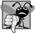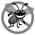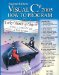# Floating-Point Numbers and Type decimal

In our next application, we depart temporarily from our GradeBook case study to declare a class called Account that maintains the balance of a bank account. Most account balances are not whole numbers (e.g., 0, 22 and 1024). For this reason, class Account represents the account balance as a real number (i.e., a number with a decimal point, such as 7.33, 0.0975 or 1000.12345). C# provides three simple types for storing real numbers in memoryfloat, double, and decimal. Types float and double are called floating-point types. The primary difference between them and decimal is that decimal variables store a limited range of real numbers precisely, whereas floating-point variables store only approximations of real numbers, but across a much greater range of values. Also, double variables can store numbers with larger magnitude and finer detail (i.e., more digits to the right of the decimal pointalso known as the number's precision) than float variables. A key application of type decimal is representing monetary amounts.

Real Number Precision and Memory Requirements

Variables of type float represent single-precision floating-point numbers and have seven significant digits. Variables of type double represent double-precision floating-point numbers. These require twice as much memory as float variables and provide 1516 significant digitsapproximately double the precision of float variables. Furthermore, variables of type decimal require twice as much memory as double variables and provide 2829 significant digits. For the range of values required by most applications, variables of type float should suffice for approximations, but you can use double or decimal to "play it safe." In some applications, even variables of type double and decimal will be inadequatesuch applications are beyond the scope of this book.

Most programmers represent floating-point numbers with type double. In fact, C# treats all real numbers you type in an application's source code (such as 7.33 and 0.0975) as double values by default. Such values in the source code are known as floating-point literals. To type a decimal literal, you must type the letter "M" or "m" at the end of a real number (for example, 7.33M is a decimal literal rather than a double). Integer literals are implicitly converted into type float, double or decimal when they are assigned to a variable of one of these types. See Appendix L, Simple Types, for the ranges of values for floats, doubles, decimals and all the other simple types.

Although floating-point numbers are not always 100% precise, they have numerous applications. For example, when we speak of a "normal" body temperature of 98.6, we do not need to be precise to a large number of digits. When we read the temperature on a thermometer as 98.6, it may actually be 98.5999473210643. Calling this number simply 98.6 is fine for most applications involving body temperatures. Due to the imprecise nature of floating-point numbers, type decimal is preferred over the floating-point types whenever the calculations need to be exact, as with monetary calculations. In cases where approximation is enough, double is preferred over type float because double variables can represent floating-point numbers more accurately. For this reason, we use type decimal throughout the book for dealing with monetary amounts and type double for other real numbers.

Real numbers also arise as a result of division. In conventional arithmetic, for example, when we divide 10 by 3, the result is 3.3333333..., with the sequence of 3s repeating infinitely. The computer allocates only a fixed amount of space to hold such a value, so clearly the stored floating-point value can be only an approximation.## Common Programming Error 4 3

Using floating-point numbers in a manner that assumes they are represented precisely can lead to logic errors.

Account Class with an Instance Variable of Type decimal

Our next application (Figs. 4.154.16) contains an oversimplified class named Account (Fig. 4.15) that maintains the balance of a bank account. A typical bank services many accounts, each with its own balance, so line 7 declares an instance variable named balance of type decimal. Variable balance is an instance variable because it is declared in the body of the class (lines 636) but outside the class's method and property declarations (lines 1013, 1619 and 2235). Every instance (i.e., object) of class Account contains its own copy of balance.

Figure 4.15. Account class with a constructor to initialize instance variable balance.

 ``` 1 // Fig. 4.15: Account.cs 2 // Account class with a constructor to 3 // initialize instance variable balance. 4 5 public class Account 6 { 7 private decimal balance; // instance variable that stores the balance 8 9 // constructor 10 public Account( decimal initialBalance ) 11 { 12 Balance = initialBalance; // set balance using property 13 } // end Account constructor 14 15 // credit (add) an amount to the account 16 public void Credit( decimal amount ) 17 { 18 Balance = Balance + amount; // add amount to balance 19 } // end method Credit 20 21 // a property to get and set the account balance 22 public decimal Balance 23 { 24 get 25 { 26 return balance; 27 } // end get 28 set 29 { 30 // validate that value is greater than or equal to 0; 31 // if it is not, balance is left unchanged 32 if ( value >= 0 ) 33 balance = value; 34 } // end set 35 } // end property Balance 36 } // end class Account ```

Figure 4.16. Create and manipulate an Account object.

(This item is displayed on pages 152 - 153 in the print version)

``` 1 // Fig. 4.16: AccountTest.cs
2 // Create and manipulate an Account object.
3 using System;
4
5 public class AccountTest
6 {
7 // Main method begins execution of C# application
8 public static void Main( string[] args )
9 {
10 Account account1 = new Account( 50.00M ); // create Account object
11 Account account2 = new Account( -7.53M ); // create Account object
12
13 // display initial balance of each object using a property
14 Console.WriteLine( "account1 balance: {0:C}",
15 account1.Balance ); // display Balance property
16 Console.WriteLine( "account2 balance: {0:C}
",
17 account2.Balance ); // display Balance property
18
```
19 decimal depositAmount; // deposit amount read from user 20 21 // prompt and obtain user input 22 Console.Write( "Enter deposit amount for account1: " ); 23 depositAmount = Convert.ToDecimal( Console.ReadLine() ); 24 Console.WriteLine( "adding {0:C} to account1 balance ", 25 depositAmount ); 26 account1.Credit( depositAmount ); // add to account1 balance 27 28 // display balances 29 Console.WriteLine( "account1 balance: {0:C}", 30 account1.Balance ); 31 Console.WriteLine( "account2 balance: {0:C} ", 32 account2.Balance ); 33 34 // prompt and obtain user input 35 Console.Write( "Enter deposit amount for account2: " ); 36 depositAmount = Convert.ToDecimal( Console.ReadLine() ); 37 Console.WriteLine( "adding {0:C} to account2 balance ", 38 depositAmount ); 39 account2.Credit( depositAmount ); // add to account2 balance 40 41 // display balances 42 Console.WriteLine( "account1 balance: {0:C}", account1.Balance ); 43 Console.WriteLine( "account2 balance: {0:C}", account2.Balance ); 44 } // end Main 45 } // end class AccountTest
 ```account1 balance: \$50.00 account2 balance: \$0.00 Enter deposit amount for account1: 49.99 adding \$49.99 to account1 balance account1 balance: \$99.99 account2 balance: \$0.00 Enter deposit amount for account2: 123.21 adding \$123.21 to account2 balance account1 balance: \$99.99 account2 balance: \$123.21 ```

Class Account contains a constructor, a method, and a property. Since it is common for someone opening an account to place money in the account immediately, the constructor (lines 1013) receives a parameter initialBalance of type decimal that represents the account's starting balance. Line 12 assigns initialBalance to the property Balance, invoking Balance's set accessor to initialize the instance variable balance.

Method Credit (lines 1619) does not return any data when it completes its task, so its return type is void. The method receives one parameter named amounta decimal value that is added to the property Balance. Line 18 uses both the get and set accessors of Balance. The expression Balance + amount invokes property Balance's get accessor to obtain the current value of instance variable balance, then adds amount to it. We then assign the result to instance variable balance by invoking the Balance property's set accessor (thus replacing the prior balance value).

Property Balance (lines 2235) provides a get accessor, which allows clients of the class (i.e., other classes that use this class) to obtain the value of a particular Account object's balance. The property has type decimal (line 22). Balance also provides an enhanced set accessor.

In Section 4.5, we introduced properties whose set accessors allow clients of a class to modify the value of a private instance variable. In Fig. 4.7, class GradeBook defines property CourseName's set accessor to assign the value received in its parameter value to instance variable courseName (line 19). This CourseName property does not ensure that courseName contains only valid data.

The application of Figs. 4.154.16 enhances the set accessor of class Account's property Balance to perform this validation (also known as validity checking). Line 32 (Fig. 4.15) ensures that value is non-negative. If the value is greater than or equal to 0, the amount stored in value is assigned to instance variable balance in line 33. Otherwise, balance is left unchanged.

AccountTest Class to Use Class Account

Class AccountTest (Fig. 4.16) creates two Account objects (lines 1011) and initializes them respectively with 50.00M and -7.53M (the decimal literals representing the real numbers 50.00 and -7.53). Note that the Account constructor (lines 1013 of Fig. 4.15) references property Balance to initialize balance. In previous examples, the benefit of referencing the property in the constructor was not evident. Now, however, the constructor takes advantage of the validation provided by the set accessor of the Balance property. The constructor simply assigns a value to Balance rather than duplicating the set accessor's validation code. When line 11 of Fig. 4.16 passes an initial balance of -7.53 to the Account constructor, the constructor passes this value to the set accessor of property Balance, where the actual initialization occurs. This value is less than 0, so the set accessor does not modify balance, leaving this instance variable with its default value of 0.

Lines 1417 in Fig. 4.16 output the balance in each Account by using the Account's Balance property. When Balance is used for account1 (line 15), the value of account1's balance is returned by the get accessor in line 26 of Fig. 4.15 and displayed by the Console.WriteLine statement (Fig. 4.16, lines 1415). Similarly, when property Balance is called for account2 from line 17, the value of the account2's balance is returned from line 26 of Fig. 4.15 and displayed by the Console.WriteLine statement (Fig. 4.16, lines 1617). Note that the balance of account2 is 0 because the constructor ensured that the account could not begin with a negative balance. The value is output by WriteLine with the format item {0:C}, which formats the account balance as a monetary amount. The : after the 0 indicates that the next character represents a format specifier, and the C format specifier after the : specifies a monetary amount (C is for currency). The cultural settings on the user's machine determine the format for displaying monetary amounts. For example, in the United States, 50 displays as \$50.00. In Germany, 50 displays as 50,00€. Figure 4.17 lists a few other format specifiers in addition to C.

Figure 4.17. string format specifiers.

Format Specifier

Description

C or c

Formats the string as currency. Precedes the number with an appropriate currency symbol (\$ in the US). Separates digits with an appropriate separator character (comma in the US) and sets the number of decimal places to two by default.

D or d

Formats the string as a decimal. Displays number as an integer.

N or n

Formats the string with commas and a default of two decimal places.

E or e

Formats the number using scientific notation with a default of six decimal places.

F or f

Formats the string with a fixed number of decimal places (two by default).

G or g

General. Formats the number normally with decimal places or using scientific notation, depending on context. If a format item does not contain a format specifier, format G is assumed implicitly.

X or x

Line 19 declares local variable depositAmount to store each deposit amount entered by the user. Unlike the instance variable balance in class Account, the local variable depositAmount in Main is not initialized to 0 by default. However, this variable does not need to be initialized here because its value will be determined by the user's input.

Line 22 prompts the user to enter a deposit amount for account1. Line 23 obtains the input from the user by calling the Console class's ReadLine method, and then passing the string entered by the user to the Convert class's ToDecimal method, which returns the decimal value in this string. Lines 2425 display the deposit amount. Line 26 calls object account1's Credit method and supplies depositAmount as the method's argument. When the method is called, the argument's value is assigned to parameter amount of method Credit (lines 1619 of Fig. 4.15), then method Credit adds that value to the balance (line 18 of Fig. 4.15). Lines 2932 (Fig. 4.16) output the balances of both Accounts again to show that only account1's balance changed.

Line 35 prompts the user to enter a deposit amount for account2. Line 36 obtains the input from the user by calling Console class's ReadLine method, and passing the return value to the Convert class's ToDecimal method. Lines 3738 display the deposit amount. Line 39 calls object account2's Credit method and supplies depositAmount as the method's argument, then method Credit adds that value to the balance. Finally, lines 4243 output the balances of both Accounts again to show that only account2's balance changed.

set and get Accessors with Different Access Modifiers

By default, the get and set accessors of a property have the same access as the propertyfor example, for a public property, the accessors are public. It is possible to declare the get and set accessors with different access modifiers. In this case, one of the accessors must implicitly have the same access as the property and the other must be declared with a more restrictive access modifier than the property. For example, in a public property, the get accessor might be public and the set accessor might be private. We demonstrate this feature in Section 9.6.## Error Prevention Tip 4 2

The benefits of data integrity are not automatic simply because instance variables are made privateyou must provide appropriate validity checking and report the errors.## Error Prevention Tip 4 3

set accessors that set the values of private data should verify that the intended new values are proper; if they are not, the set accessors should leave the instance variables unchanged and generate an error. We demonstrate how to gracefully generate errors in Chapter 12, Exception Handling.

UML Class Diagram for Class Account

The UML class diagram in Fig. 4.18 models class Account of Fig. 4.15. The diagram models the Balance property as a UML attribute of type decimal (because the corresponding C# property had type decimal). The diagram models class Account's constructor with a parameter initialBalance of type decimal in the third compartment of the class. The diagram models operation Credit in the third compartment with an amount parameter of type decimal (because the corresponding method has an amount parameter of C# type decimal).

Figure 4.18. UML class diagram indicating that class Account has a public Balance property of type decimal, a constructor and a method.### (Optional) Software Engineering Case Study Identifying the Classes in the ATM Requirements DocumentVisual C# 2005 How to Program (2nd Edition)
ISBN: 0131525239
EAN: 2147483647
Year: 2004
Pages: 600